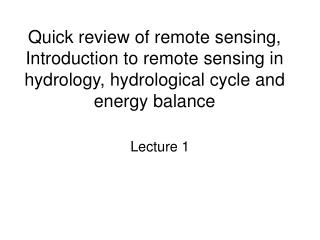DownloadDownload PresentationQuick review of remote sensing, Introduction to remote sensing in hydrology, hydrological cycle and energy balance

# Quick review of remote sensing, Introduction to remote sensing in hydrology, hydrological cycle and energy balance

Download Presentation## Quick review of remote sensing, Introduction to remote sensing in hydrology, hydrological cycle and energy balance

- - - - - - - - - - - - - - - - - - - - - - - - - - - E N D - - - - - - - - - - - - - - - - - - - - - - - - - - -
##### Presentation Transcript

1. Quick review of remote sensing, Introduction to remote sensing in hydrology, hydrological cycle and energy balance Lecture 1

2. Hydrology focuses on the global hydrologic cycle and the processes involved in the land phase of that cycle. Hydrology describes and predicts: The spatial and temporal variations of water substance in the terrestrial, oceanic, and atmospheric compartments of the global water system. The movement of water on and under the earth’s surface, the physical, chemical, and biological processes accompanying that movement.

3. Historical Benchmarks Monitoring of river flows started by Egyptians 3800 B. P. Concept of hydrologic cycle, 3000 B. P., King Solomon in Ecclesiastes: All the rivers run into the sea; ….. unto the place from whence the rivers come, thither they return again. Rainfall measuring, 2400 B. P. in India. . . . . Formal recognition of the scientific status of hydrology, 1920’s and 1930’s, UGG, AGU…etc.

4. 1.Hydrologic cycle All units in the picture above are 1012 m3. http://www.globalchange.umich.edu/globalchange1/current/labs/water_cycle/water_cycle.html exercises • Identify the reservoirs of the hydrological cycle • Hydrological processes (flux) connecting the reservoirs • Write the water balance equations for ocean, atmosphere, and land.

5. Reservoirs http://ww2010.atmos.uiuc.edu/(Gh)/guides/mtr/hyd/bdgt.rxml

6. Hornberger et al., 1998

7. Water Storage in the Atmosphere 0.001% Water vapor Clouds (water vapor condensed on particulate)

8. Water Balance Equation where: M = mass within the control volume [M]; t = time [T]; I' = mass inflow rate [M T-1]; and O' = mass outflow rate [M T-1]. where: V = volume of water within the control volume [L3]; I = volume inflow rate [L3 T-1]; and O = volume outflow rate [L3 T-1].

9. where: V = average volume of water stored, and assumed to be constant; P = average precipitation rate; rsi= average surface water inflow rate; rgi= average groundwater inflow rate; rso= average surface water outflow rate; rgo= average groundwater outflow rate; and et = average evapotranspiration rate. All terms in the equation have dimensions of volume per time [L3 T-1].

10. What drives the hydrologic cycle?

11. 2. Energy Balance http://geography.otago.ac.nz/Courses/283_389/Resources/palaeo/GlobalEnergyBalance.html exercises • Write the energy balance equations for the earth

12. Short-wave radiation http://www.physicalgeography.net/fundamentals/7i.html

13. Campbell and Norman, 1998

14. Long-wave radiation http://www.physicalgeography.net/fundamentals/7i.html exercises • Write the energy balance equations for the earth surface

15. Campbell and Norman, 1998

16. Discussion • What determine incident short-wave radiation on the earth surface? • Solar constant: Describes the Solar Radiation that falls on an area above the atmosphere at a vertical angle: s = 1.37 kW / m². • others • What control out-going long-wave radiation from the earth surface?

17. Describe the patterns, and Explain… Find the difference between the two http://www.agu.org/eos_elec/95206e.html

18. How is the Hydrological Cycle driven?

19. 3. Atmospheric circulations If no earth rotation With earth rotation http://ess.geology.ufl.edu/ess/Notes/AtmosphericCirculation/atmosphere.html

20. http://www.blueplanetbiomes.org/climate.htm

21. 4. Oceanic circulations http://www.uwsp.edu/geo/faculty/ritter/images/maps/ocean_currents.jpg

22. Hydrologic Information System to the Statein (Near) Real Time Via Internet • Products: • - Rainfall • - Snow cover • - Runoff • - River flow • - Recharge • - ET • Soil Moisture • LULC • VI